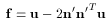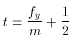# glTexGen

### NAME

glTexGend, glTexGenf, glTexGeni, glTexGendv, glTexGenfv, glTexGeniv -- control the generation of texture coordinates

### C SPECIFICATION

```void glTexGend(GLenum coord,
GLenum pname,
GLdouble param)
void glTexGenf(GLenum coord,
GLenum pname,
GLfloat param)
void glTexGeni(GLenum coord,
GLenum pname,
GLint param)
```

### PARAMETERS

coord
Specifies a texture coordinate. Must be one of the following: GL_S, GL_T, GL_R, or GL_Q.

pname
Specifies the symbolic name of the texture-coordinate generation function. Must be GL_TEXTURE_GEN_MODE.

param
Specifies a single-valued texture generation parameter, one of GL_OBJECT_LINEAR, GL_EYE_LINEAR, or GL_SPHERE_MAP.

### C SPECIFICATION

```void glTexGendv(GLenum coord,
GLenum pname,
const GLdouble *params)
void glTexGenfv(GLenum coord,
GLenum pname,
const GLfloat *params)
void glTexGeniv(GLenum coord,
GLenum pname,
const GLint *params)
```

### PARAMETERS

coord
Specifies a texture coordinate. Must be one of the following: GL_S, GL_T, GL_R, or GL_Q.

pname
Specifies the symbolic name of the texture-coordinate generation function or function parameters. Must be GL_TEXTURE_GEN_MODE, GL_OBJECT_PLANE, or GL_EYE_PLANE.

params
Specifies a pointer to an array of texture generation parameters. If pname is GL_TEXTURE_GEN_MODE, then the array must contain a single symbolic constant, one of GL_OBJECT_LINEAR, GL_EYE_LINEAR, or GL_SPHERE_MAP. Otherwise, params holds the coefficients for the texture-coordinate generation function specified by pname.

### DESCRIPTION

glTexGen selects a texture-coordinate generation function or supplies coefficients for one of the functions. coord names one of the (s, t, r, q) texture coordinates, and it must be one of these symbols: GL_S, GL_T, GL_R, or GL_Q. pname must be one of three symbolic constants: GL_TEXTURE_GEN_MODE, GL_OBJECT_PLANE, or GL_EYE_PLANE. If pname is GL_TEXTURE_GEN_MODE, then params chooses a mode, one of GL_OBJECT_LINEAR, GL_EYE_LINEAR, or GL_SPHERE_MAP. If pname is either GL_OBJECT_PLANE or GL_EYE_PLANE, params contains coefficients for the corresponding texture generation function.

If the texture generation function is GL_OBJECT_LINEAR, the function

g = p1 * xo + p2 * yo + p3 * zo + p4 * wo

is used, where g is the value computed for the coordinate named in coord, p1, p2, p3, and p4 are the four values supplied in params, and xo, yo, zo, and wo are the object coordinates of the vertex. This function can be used to texture-map terrain using sea level as a reference plane (defined by p1, p2, p3, and p4). The altitude of a terrain vertex is computed by the GL_OBJECT_LINEAR coordinate generation function as its distance from sea level; that altitude is used to index the texture image to map white snow onto peaks and green grass onto foothills, for example.

If the texture generation function is GL_EYE_LINEAR, the function

g =p1' * xe + p2' * ye + p3' * ze + p4' * we

is used, where

(p1' p2' p3' p4') = (p1 p2 p3 p4) * M^-1

and xe, ye, ze, and we are the eye coordinates of the vertex, p1, p2, p3, and p4 are the values supplied in params, and M is the modelview matrix when glTexGen is invoked. If M is poorly conditioned or singular, texture coordinates generated by the resulting function may be inaccurate or undefined.

Note that the values in params define a reference plane in eye coordinates. The modelview matrix that is applied to them may not be the same one in effect when the polygon vertices are transformed. This function establishes a field of texture coordinates that can produce dynamic contour lines on moving objects.

If pname is GL_SPHERE_MAP and coord is either GL_S or GL_T, s and t texture coordinates are generated as follows. Let u be the unit vector pointing from the origin to the polygon vertex (in eye coordinates). Let n' be the current normal, after transformation to eye coordinates. Let f = (fx fy fz)^T be the reflection vector such thatFinally, let m = 2(fx^2 + fy^2 + (fz + 1)^2)^(1/2). Then the values assigned to the s and t texture coordinates areA texture-coordinate generation function is enabled or disabled using glEnable or glDisable with one of the symbolic texture-coordinate names (GL_TEXTURE_GEN_S, GL_TEXTURE_GEN_T, GL_TEXTURE_GEN_R, or GL_TEXTURE_GEN_Q) as the argument. When enabled, the specified texture coordinate is computed according to the generating function associated with that coordinate. When disabled, subsequent vertices take the specified texture coordinate from the current set of texture coordinates. Initially, all texture generation functions are set to GL_EYE_LINEAR and are disabled. Both s plane equations are (1, 0, 0, 0), both t plane equations are (0, 1, 0, 0), and all r and q plane equations are (0, 0, 0, 0).

### ERRORS

GL_INVALID_ENUM is generated when coord or pname is not an accepted defined value, or when pname is GL_TEXTURE_GEN_MODE and params is not an accepted defined value.

GL_INVALID_ENUM is generated when pname is GL_TEXTURE_GEN_MODE, params is GL_SPHERE_MAP, and coord is either GL_R or GL_Q.

GL_INVALID_OPERATION is generated if glTexGen is called between a call to glBegin and the corresponding call to glEnd.

### ASSOCIATED GETS

glGetTexGen
glIsEnabled with argument GL_TEXTURE_GEN_S
glIsEnabled with argument GL_TEXTURE_GEN_T
glIsEnabled with argument GL_TEXTURE_GEN_R
glIsEnabled with argument GL_TEXTURE_GEN_Q